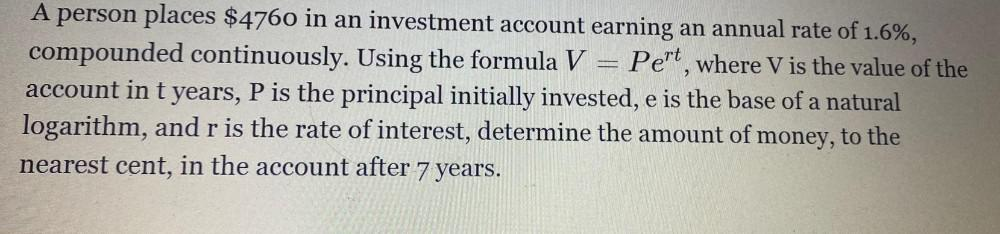Question:

# 2 A person places \$4760 in an investment account earning an annual rate of 1.6%, compounded continuously. Using the formula V =2 A person places \$4760 in an investment account earning an annual rate of 1.6%, compounded continuously. Using the formula V = Pert, where V is the value of the account in t years, P is the principal initially invested, e is the base of a natural logarithm, and r is the rate of interest, determine the amount of money, to the nearest cent, in the account after 7 years. a